# CBSE Class 12 Physics Chapter 9 Extra Questions## myCBSEguide App

Complete Guide for CBSE Students

NCERT Solutions, NCERT Exemplars, Revison Notes, Free Videos, CBSE Papers, MCQ Tests & more.

CBSE Class 12 Physics Chapter 9 Extra Questions. We know Physics is tough subject within the consortium of science subjects physics is an important subject. But if you want to make career in these fields like IT Consultant, Lab Technician, Laser Engineer, Optical Engineer etc. You need to have strong fundamentals in physics to crack the exam. myCBSEguide has just released Chapter Wise Question Answers for class 12 Physics. There chapter wise Extra Questions with complete solutions are available for download in myCBSEguide website and mobile app. These Questions with solution are prepared by our team of expert teachers who are teaching grade in CBSE schools for years. There are around 4-5 set of solved Physics Extra Questions from each and every chapter. The students will not miss any concept in these Chapter wise question that are specially designed to tackle Board Exam. We have taken care of every single concept given in CBSE Class 12 Physics syllabus and questions are framed as per the latest marking scheme and blue print issued by CBSE for class 12.

Class 12 Physics Chapter 9 Extra Questions

## Important Questions for Class 12 Physics Ray Optics and Optical

Class – 12 Physics (Ray Optics and Optical Instruments)

1. According to Cartesian sign convention the heights measured

1. leftwards with respect to x-axis and normal to the principal axis (x-axis) of the mirror/lens are taken as positive
2. downwards with respect to x-axis and normal to the principal axis (x-axis) of the mirror/lens are taken as positive
3. rightwards with respect to x-axis and normal to the principal axis (x-axis) of the mirror/lens are taken as positive
4. upwards with respect to principal axis (x-axis) and normal to the principal axis (x-axis) of the mirror/lens are taken as positive
2. A lens of power + 2.0 D is placed in contact with another lens of power – 1.0 D. The combination will behave like
1. a converging lens of focal length 100 cm
2. a diverging lens of focal length 50 cm
3. a converging lens of focal length 50 cm
4. a diverging lens of focal length 100 cm
3. Dispersion is
1. The phenomenon of scattering of light from various molecules
2. The variation of refractive index from glass to glass
3. The phenomenon of splitting of light into electric and magnetic fields
4. The phenomenon of splitting of light into its component colours
4. A converging lens is used to form an image on a screen. When the upper half of the lens is covered by an opaque screen
1. none of these
2. complete image will be formed
3. intensity of the image will increase
4. half the image will disappear
5. Total internal reflection can take place only if
1. incidence angle = {tex}\frac{1}{{{n_{21}}}}{/tex}, {tex}{{\rm{n}}_{{\rm{21}}}}{/tex} = refractive index of medium 2 w.r.t. medium 1
2. tan(incidence angle) > {tex}\frac{1}{{{n_{21}}}}{/tex}, {tex}{{\rm{n}}_{{\rm{21}}}}{/tex} = refractive index of medium 2 w.r.t. medium 1
3. cos(incidence angle) ={tex}\frac{1}{{{n_{21}}}}{/tex} 1, {tex}{{\rm{n}}_{{\rm{21}}}}{/tex} = refractive index of medium 2 w.r.t. medium 1
4. sin(incidence angle) > {tex}\frac{1}{{{n_{21}}}}{/tex}, n21=refractive index of medium 2 w.r.t. medium 1
6. What is the number of images of an object held between two parallel plane mirrors?

7. How does the angle of minimum deviation of a glass prism vary, if the incident violet light is replaced with red light?

8. Write down expression for its magnifying power.

9. Write the relation for the refractive index {tex}\mu {/tex} of the prism in terms of the angle of minimum deviation {tex}\delta m{/tex} and the angle A of prism.

10. Draw a labeled ray diagram of a reflecting type telescope. Write its any one advantage over refracting type telescope.

11. How does the power of a convex lens vary, if the incident red light replaced by violet light?

12. A ray of light incident at 49° on the face of an equilateral prism passes symmetrically. Calculate the refractive index of the material of the prism.

13. The near point of a hypemetropic person is 50 cm from the eye. What is the power of the lens required to enable the person to read clearly a book held at 25 cm from the eye?

14. Two thin lenses, both of 10 cm focal length one convex and other concave, are placed 5 cm apart. An object is placed 20 cm in front of the convex lens. Find the nature and position of the final image.
15. A prism is found to give a minimum deviation of 51°. The same prism gives a deviation of 62°48′ for two values of the angles of incidence, namely, 46°6′ and 82°42′. Determine the refractive angle of the prism and the refractive index of its material.

Class – 12 Physics (Ray Optics and Optical Instruments)

1. upwards with respect to principal axis (x-axis) and normal to the principal axis (x-axis) of the mirror/lens are taken as positive
Explanation: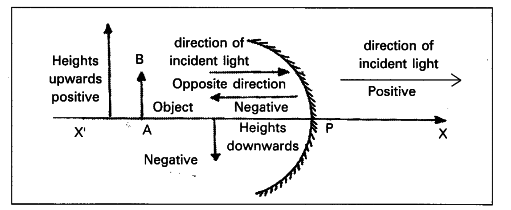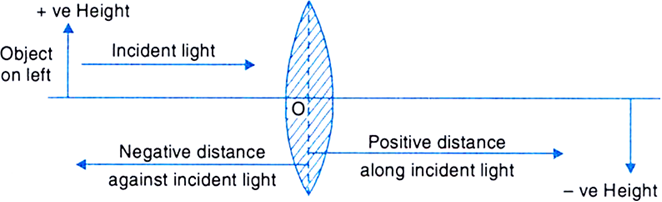1. a converging lens of focal length 100 cm
Explanation: Combined power, P = P1 + P2 = +2 D + (-1 D) = +1 D
focal length of combination, f = 1/P = 1/1 = 1m = =100 cm
Since focal length is positive, it will behave as converging lens.
1. The phenomenon of splitting of light into its component colours
Explanation: In dispersion, light of different wavelength deviates by different angles, hence it splits into consituent colors.
1. complete image will be formed
Explanation: complete image will be formed at same position as the light rays passing from the transparent portion will still converge at the same points. But less number of rays passes through the lens, hence intensity of image decreases.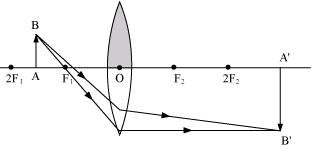1. sin(incidence angle) > {tex}\frac{1}{{{n_{21}}}}{/tex}, n21=refractive index of medium 2 w.r.t. medium
Explanation: n21 = refractive index of medium 2 w.r.t. medium 1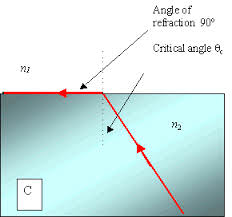According to Snell’s law, {tex}{sini\over sinr}={n_1\over n_2}=n_{12}={1\over n_{21}}{/tex}
When i = c, r = 90°
Hence sinc = {tex}\frac{1}{{{n_{21}}}}{/tex}
For TIR to take place, i > c hence sin i > sinc (i.e. {tex}\frac{1}{{{n_{21}}}}{/tex})
1. Given {tex}\theta = 0^\circ{/tex}
Number of images {tex}n = \frac{{360^\circ }}{\theta } – 1{/tex}
{tex}= \frac{{360^\circ }}{{0^\circ }} – 1{/tex} = infinite
2. {tex}\because {\delta _{violet}} > {\delta _{red}}{/tex}
When the incident violet light is replaced with red light, the angle of minimum deviation of a glass decreases.
3. Magnifying power, {tex}m = \frac{{{F_0}}}{{{F_e}}}\left( {1 + \frac{{{F_e}}}{D}} \right){/tex}
4. {tex}\mu = \frac{{\sin \left( {\frac{{A + {\delta _m}}}{2}} \right)}}{{\sin \frac{A}{2}}}{/tex}
5. The ray diagram of a reflecting type telescope is shown below: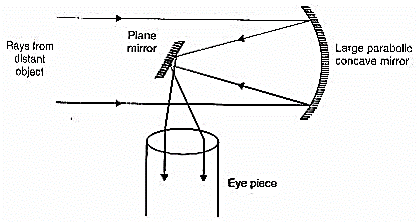Since a reflecting telescope has mirror objective, the image formed is free from chromatic aberration.
6. By the lens maker’s formula
{tex}\frac{1}{f} = (\mu – 1)\left( {\frac{1}{{{R_1}}} – \frac{1}{{{R_2}}}} \right){/tex}
Power of lens {tex}P = \frac{1}{f} = (\mu – 1)\left( {\frac{1}{{{R_1}}} – \frac{1}{{{R_2}}}} \right){/tex}
{tex}\because {\mu _{violet}} > {\mu _{red}}{/tex}
{tex}\therefore{/tex} Power of the lens will be increased.
7. As the prism is an equilateral one, A = 60°. Since the ray of light passes symmetrically, the prism is in the position of minimum deviation.
So, {tex}r = \frac{A}{2} = \frac{{60^\circ }}{2} = 30^\circ {/tex}
Also i = 49°
{tex}\therefore \mu = \frac{{\sin i}}{{\sin r}} = \frac{{\sin 49^\circ }}{{\sin 30^\circ }} = \frac{{0.7547}}{{0.5}}{/tex}
or {tex}\mu = 1.5{/tex}
8. Given, u = -25cm, v = -50 cm, f = ?
Using lens formula,
{tex}\frac{1}{f} = \frac{1}{v} – \frac{1}{u} = \frac{1}{{ – 50}} – \frac{1}{{ – 25}} = – \frac{1}{{50}} + \frac{1}{{25}}{/tex}
{tex} = \frac{{ – 1 + 2}}{{50}} = \frac{1}{{50}}{/tex}
{tex}\therefore{/tex}f = 50 cm
{tex}P = \frac{1}{{f(in\;m)}} = \frac{{100}}{{f(in\;cm)}}{/tex}
{tex} \Rightarrow P = \frac{{100}}{{50}} = + 2D{/tex}
Hence, the corrective lens is convex.
9.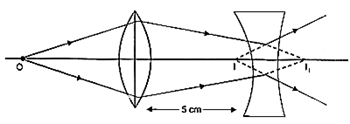For refraction at the convex lens, we have
u = -20 cm; f1 = 10 cm; v = v1 =?
Using lens formula, we have
{tex}\frac{1}{{{v_1}}} – \frac{1}{{( – 20)}} = \frac{1}{{10}}{/tex}
{tex} \Rightarrow {/tex}v1 = 0 + 20 cm
The convex lens produces converging rays trying to meet at I1, 20 cm from the convex lens, i.e. 15 cm behind the concave lens.
I1 will serve as a vertical object for the concave lens.
For refraction at the concave lens, we have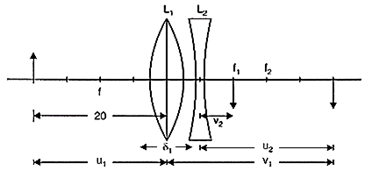For concave lens,
u = 20 – 5 = 15 cm, f = -10 cm
As per sign convention
u = -15
f = -10
{tex}\frac{1}{f} = \frac{1}{v} + \frac{1}{u}{/tex}
{tex}\frac{1}{v} = \frac{1}{f} + \frac{1}{u}{/tex}
{tex} = – \frac{1}{{10}} – \frac{1}{{15}}{/tex}
{tex} = \frac{{ – 3 – 2}}{{30}} = \frac{{ – 6}}{{30}}{/tex}
{tex}\frac{1}{v} = – \frac{1}{5}{/tex}
v = -5 cm
i.e. This image is in the side of object 5 cm Right to concave and 10 cm (5 + 5) from convex
u = + 15 cm v = ? , f = -10cm
Using lens formula, we have
{tex}\frac{1}{v} – \frac{1}{{15}} = – \frac{1}{{10}}{/tex}
v = -30 cm
Hence, the final image is virtual and is located at 30 cm to the left of the concave lens.
10. The incident ray is deviated through {tex}\delta = 62^\circ 48′{/tex} when angle i = 40o6′. From the principle of reversibility of light, it is clear from the figure that the emergent ray (for which angle e = 82o42′) is also deviated through the same angle {tex}\delta {/tex}. Now,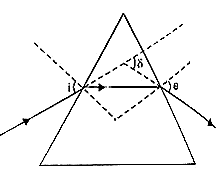{tex}\delta = (i + e) – A{/tex}
or {tex}A = (i + e) – \delta {/tex}
{tex} = 40^\circ 6′ + 82^\circ 42′ – 62^\circ 48′{/tex}
or A = 60°
which is the refractive angle of the prism.
For minimum deviation, i = e
Hence, {tex}{\delta _{\min }} = 2i – A{/tex}
or {tex}i = \left( {\frac{{{\delta _{\min }} + A}}{2}} \right){/tex}
{tex} = \frac{{(51^\circ + 60^\circ )}}{2} = 55^\circ 30′{/tex}
Which is the angle of incidence at minimum deviation? The refractive index of the material of the prism is given by
{tex}\mu = \frac{{\sin \frac{{({\delta _{\min }} + A)}}{2}}}{{\sin \frac{A}{2}}}{/tex}
or {tex}\mu = \frac{{\sin \left( {\frac{{51^\circ + 60^\circ }}{2}} \right)}}{{\sin \frac{{60^\circ }}{2}}}{/tex}
or {tex}\mu = 1.648{/tex}

## Chapter Wise Extra Questions of Class 12 Physics Part I & Part II## Test Generator

Create Papers with your Name & Logo